# GMAT Math : DSQ: Solving linear equations with two unknowns

## Example Questions

← Previous 1

### Example Question #1 : Linear Equations, Two Unknowns

What is the value of z?

Statement 1: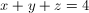Statement 2: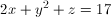Possible Answers:

EACH statement ALONE is sufficient.

Statement 2 ALONE is sufficient, but statement 1 is not sufficient.

Statement 1 ALONE is sufficient, but statement 2 is not sufficient.

BOTH statements TOGETHER are sufficient, but NEITHER statement ALONE is sufficient.

Statements 1 and 2 TOGETHER are NOT sufficient.

Correct answer:

Statements 1 and 2 TOGETHER are NOT sufficient.

Explanation:

To solve for three variables, you must have three equations. Statements 1 and 2 together only give two equations, so the statements together are not sufficient.

### Example Question #1 : Dsq: Solving Linear Equations With Two Unknowns

Is the equation linear?

Statement 1: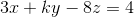Statement 2:is a constant

Possible Answers:

Statement 1 ALONE is sufficient, but statement 2 is not sufficient.

BOTH statements TOGETHER are sufficient, but NEITHER statement ALONE is sufficient.

Statement 2 ALONE is sufficient, but statement 1 is not sufficient.

Statements 1 and 2 TOGETHER are NOT sufficient.

EACH statement ALONE is sufficient.

Correct answer:

BOTH statements TOGETHER are sufficient, but NEITHER statement ALONE is sufficient.

Explanation:

If we only look at statement 1, we might think the equation is not linear because of theterm.  But statement 2 tells us theis a constant.  Then the equation is linear.  We need both statements to answer this question.

### Example Question #1 : Linear Equations, Two Unknowns

Data sufficiency question- do not actually solve the question

Solve for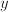: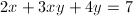1.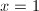2.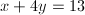Possible Answers:

Statements 1 and 2 together are not sufficient, and additional data is needed to answer the question

Statement 1 alone is sufficient but statement 2 alone is not sufficient to answer the question

Each statement alone is sufficient

Both statements taken together are sufficient to answer the question, but neither question alone is sufficient

Statement 2 alone is sufficient but statement 1 alone is not sufficient to answer the question

Correct answer:

Each statement alone is sufficient

Explanation:

When solving an equation with 2 variables, a second equation or the solution of 1 variable is necessary to solve.

### Example Question #4 : Linear Equations, Two Unknowns

Data Sufficiency Question

Solve forand.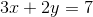1.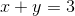2. Bothandare positive integers

Possible Answers:

both statements taken together are sufficient to answer the question, but neither statement alone is sufficient

statement 1 alone is sufficient, but statement 2 alone is not sufficient to answer the question

statement 2 alone is sufficient, but statement 1 alone is not sufficient to answer the question

statements 1 and 2 together are not sufficient, and additional data is needed to answer the question

each statement alone is sufficient

Correct answer:

each statement alone is sufficient

Explanation:

Using statement 1 we can set up a series of equations and solve for bothand.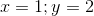Additionally, the information in statement 2 indicates that there is only one possible solution that satisfies the requirement that both are positive integers.

### Example Question #2 : Linear Equations, Two Unknowns

Given that both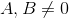, how many solutions does this system of equations have: one, none, or infinitely many?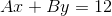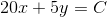Statement 1: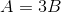Statement 2: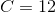Possible Answers:

Statement 1 ALONE is sufficient to answer the question, but Statement 2 ALONE is NOT sufficient to answer the question.

BOTH statements TOGETHER are sufficient to answer the question, but NEITHER statement ALONE is sufficient to answer the question.

Statement 2 ALONE is sufficient to answer the question, but Statement 1 ALONE is NOT sufficient to answer the question.

EITHER statement ALONE is sufficient to answer the question.

BOTH statements TOGETHER are insufficient to answer the question.

Correct answer:

Statement 1 ALONE is sufficient to answer the question, but Statement 2 ALONE is NOT sufficient to answer the question.

Explanation:

If the slopes of the lines are not equal, then the lines intersect at one solution. If the slopes are equal, then there are two possibilties: either they do not intersect or they are the same line. Write each equation in slope-intercept form: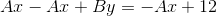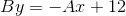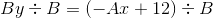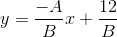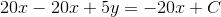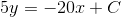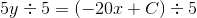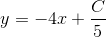The slopes of these lines are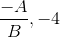.

If Statement 1 is true, then we can rewrite the first slope as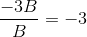, meaning that the lines have unequal slopes, and that there is only one solution. Statement 2 tells us the value of, which is irrelevant.

### Example Question #1 : Dsq: Solving Linear Equations With Two Unknowns

How many solutions does this system of equations have: one, none, or infinitely many?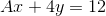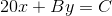Statement 1: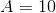Statement 2: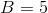Possible Answers:

BOTH statements TOGETHER are sufficient to answer the question, but NEITHER statement ALONE is sufficient to answer the question.

Statement 2 ALONE is sufficient to answer the question, but Statement 1 ALONE is NOT sufficient to answer the question.

EITHER statement ALONE is sufficient to answer the question.

BOTH statements TOGETHER are insufficient to answer the question.

Statement 1 ALONE is sufficient to answer the question, but Statement 2 ALONE is NOT sufficient to answer the question.

Correct answer:

BOTH statements TOGETHER are sufficient to answer the question, but NEITHER statement ALONE is sufficient to answer the question.

Explanation:

If the slopes of the lines are not equal, then the lines intersect at one solution; if they are equal, then they do not intersect, or the lines are the same line. Write each equation in slope-intercept form,: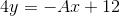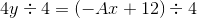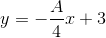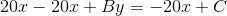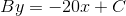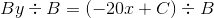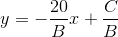The slopes of the lines are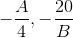.

We need to know bothandin order to determine their equality or inequality, and only if they are unequal can we answer the question.

Setand.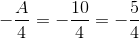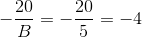The slopes are unequal, so the lines intersect at one point; the system has exactly one solution.

### Example Question #2 : Dsq: Solving Linear Equations With Two Unknowns

Solve the following for x:

4x+7y = 169

1. x > y

2. x - y = 12

Possible Answers:

Statements 1 and 2 together are not sufficient.

Statement 1 alone is sufficient, but statement 2 alone is not sufficient to answer the question.

Each statement alone is sufficient.

Statement 2 alone is sufficient, but statement 1 alone is not sufficient to answer the question.

Both statements taken together are sufficient to answer the question, but neither statement alone is sufficient.

Correct answer:

Statement 2 alone is sufficient, but statement 1 alone is not sufficient to answer the question.

Explanation:

To solve with 2 unknowns, we must create a system of equations with at least 2 equations. Using statement 2 as a second equation we can easily get our answer. Solve statement 2 for x or y, and plug in for the corresponding variable in the equation given by the problem.

So, solving statement 2 for x, we get x=12+y. Replacing x in the equation from the problem, we get 4(12+y) + 7y=169. We can distribute the 4, and combine terms to find 48+11y=169. Subtract 48 from both sides, we get 11y=121. So y=11. Reusing either equation and plugging in our y value gives our x value. So x - 11=12, or x=23. This shows that x > y, and statement 1 is true. But even though it's true, it is completely unneccessary information. Therefore the answer is that we only need the information from statement 2, and statement 1 is not needed.

### Example Question #8 : Linear Equations, Two Unknowns

Given that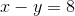, evaluate.

Statement 1: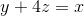Statement 2: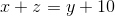Possible Answers:

BOTH statements TOGETHER are insufficient to answer the question.

Statement 2 ALONE is sufficient to answer the question, but Statement 1 ALONE is NOT sufficient to answer the question.

BOTH statements TOGETHER are sufficient to answer the question, but NEITHER statement ALONE is sufficient to answer the question.

Statement 1 ALONE is sufficient to answer the question, but Statement 2 ALONE is NOT sufficient to answer the question.

EITHER statement ALONE is sufficient to answer the question.

Correct answer:

EITHER statement ALONE is sufficient to answer the question.

Explanation:

Solve forin each statement.

Statement 1: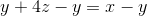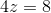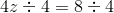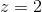Statement 2: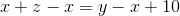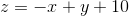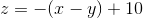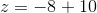From either statement alone, it can be deduced that.

### Example Question #3 : Linear Equations, Two Unknowns

Data Sufficiency Question

Solve forand:1.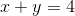2.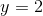Possible Answers:

Both statements taken together are sufficient to answer the question, but neither statement alone is sufficient

Each statement alone is sufficient

Statement 2 alone is sufficient, but statement 1 alone is not sufficient to answer the question

Statements 1 and 2 together are not sufficient, and additional data is needed to answer the question

Statement 1 alone is sufficient, but statement 2 alone is not sufficient to answer the question

Correct answer:

Each statement alone is sufficient

Explanation:

In order to solve an equation set, one requires a number of equations equal to the number of variables. Therefore, either of the statements allow the problem to be solved.

### Example Question #10 : Linear Equations, Two Unknowns

Data Sufficiency Question

Solve for,, and: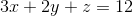1.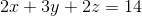2.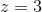Possible Answers:

Statement 1 alone is sufficient, but statement 2 alone is not sufficient to answer the question

Statements 1 and 2 together are not sufficient, and additional data is needed to answer the question

Each statement alone is sufficient

Statement 2 alone is sufficient, but statement 1 alone is not sufficient to answer the question

Both statements taken together are sufficient to answer the question, but neither statement alone is sufficient

Correct answer:

Both statements taken together are sufficient to answer the question, but neither statement alone is sufficient

Explanation:

In order to solve an equation set, one requires a number of equations equal to the number of variables. Therefore, three equations are needed and both statements are required to solve the problem.

← Previous 1

### All GMAT Math Resources